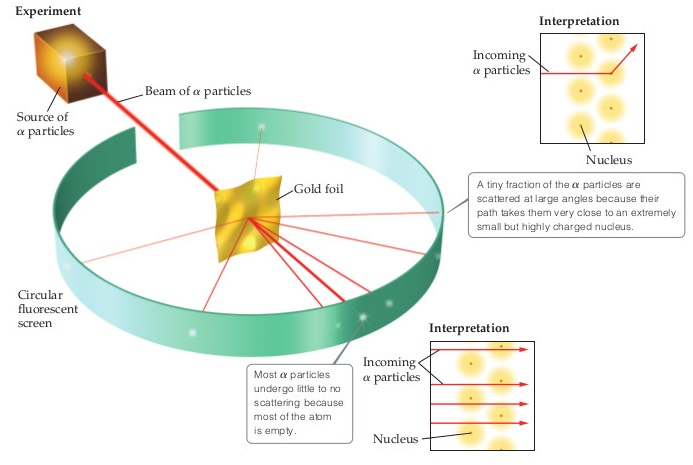# Problem: You may want to reference (Pages 46 - 49) Section 2.2 while completing this problem.What fraction of the α particles in Rutherford's gold foil experiment are scattered at large angles? Assume the gold foil is two layers thick, as shown in the figure, and that the approximate diameters of a gold atom and its nucleus are 2.2 Å and 1.0 × 10− 4 Å, respectively. Hint: Calculate the cross-sectional area occupied by the nucleus as a fraction of that occupied by the atom. Assume that the gold nuclei in each layer are offset from each other.

###### FREE Expert Solution

90% (376 ratings)###### Problem Details

You may want to reference (Pages 46 - 49) Section 2.2 while completing this problem.What fraction of the α particles in Rutherford's gold foil experiment are scattered at large angles? Assume the gold foil is two layers thick, as shown in the figure, and that the approximate diameters of a gold atom and its nucleus are 2.2 Å and 1.0 × 10− 4 Å, respectively. Hint: Calculate the cross-sectional area occupied by the nucleus as a fraction of that occupied by the atom. Assume that the gold nuclei in each layer are offset from each other.

Frequently Asked Questions

What scientific concept do you need to know in order to solve this problem?

Our tutors have indicated that to solve this problem you will need to apply the Rutherford Gold Foil Experiment concept. You can view video lessons to learn Rutherford Gold Foil Experiment. Or if you need more Rutherford Gold Foil Experiment practice, you can also practice Rutherford Gold Foil Experiment practice problems.

What professor is this problem relevant for?

Based on our data, we think this problem is relevant for Professor Sharma's class at HCC.